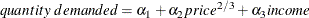# The MODEL Procedure

### Goal Seeking: Solving for Right-Hand-Side Variables

The process of computing input values that are needed to produce target results is often called goal seeking. To compute a goal-seeking solution, use a SOLVE statement that lists the variables you want to solve for and provide a data set that contains values for the remaining variables.

Consider the following demand model for packaged ricewhere price is the price of the package and income is disposable personal income. The only variable the company has control over is the price it charges for rice. This model is estimated by using the following simulated data and PROC MODEL statements:

data demand;
do t=1 to 40;
price = (rannor(10) +5) * 10;
income = 8000 * t ** (1/8);
demand = 7200 - 1054 * price ** (2/3) +
7 * income + 100 * rannor(1);
output;
end;
run;

data goal;
demand = 85000;
income = 12686;
run;


The goal is to find the price the company would have to charge to meet a sales target of 85,000 units. To do this, a data set is created with a DEMAND variable set to 85000 and with an INCOME variable set to 12686, the last income value.

The desired price is then determined by using the following PROC MODEL statements:

proc model data=demand
outmodel=demandModel;
demand = a1 - a2 * price ** (2/3) + a3 * income;
fit demand / outest=demest;
solve price / estdata=demest data=goal solveprint;
run;


The SOLVEPRINT option prints the solution values, number of iterations, and final residuals at each observation. The SOLVEPRINT output from this solve is shown in Figure 19.82.

Figure 19.82: Goal Seeking, SOLVEPRINT Output

The MODEL Procedure
Single-Equation Simulation

 Observation Iterations CC ERROR.demand 1 6 0 0

Solution Values
price
33.59016

The output indicates that it took six Newton iterations to determine the PRICE of 33.5902, which makes the DEMAND value within 16E–11 of the goal of 85,000 units.

Consider a more ambitious goal of 100,000 units. The output shown in Figure 19.83 indicates that the sales target of 100,000 units is not attainable according to this model.

data goal;
demand = 100000;
income = 12686;
run;

proc model model=demandModel;
solve price / estdata=demest data=goal solveprint;
run;


Figure 19.83: Goal Seeking, Convergence Failure

The MODEL Procedure
Single-Equation Simulation

 Could not reduce norm of residuals in 10 subiterations.

 The solution failed because 1 equations are missing or have extreme values for observation 1 at NEWTON iteration 1.

Observation Iteration CC 1 1 -1.000000 1

  The MODEL Procedure Single-Equation Simulation --- Listing of Program Data Vector --- _N_: 12 ACTUAL.demand: 100000 ERROR.demand: . PRED.demand: . a1: 7126.437997 a2: 1040.841492 a3: 6.992694 demand: 100000 income: 12686 price: -0.000172 @PRED.demand/@pri: . 

The program data vector with the error note indicates that even after 10 subiterations, the norm of the residuals could not be reduced. The sales target of 100,000 units are unattainable with the given model. You might need to reformulate your model or collect more data to more accurately reflect the market response.